# Solving Quadratic Equations 8 6 by Factoring Warm

• Slides: 16Solving Quadratic Equations 8 -6 by Factoring Warm Up Lesson Presentation Lesson Quiz Holt Mc. Dougal Algebra 1 Algebra 11 Holt Mc. Dougal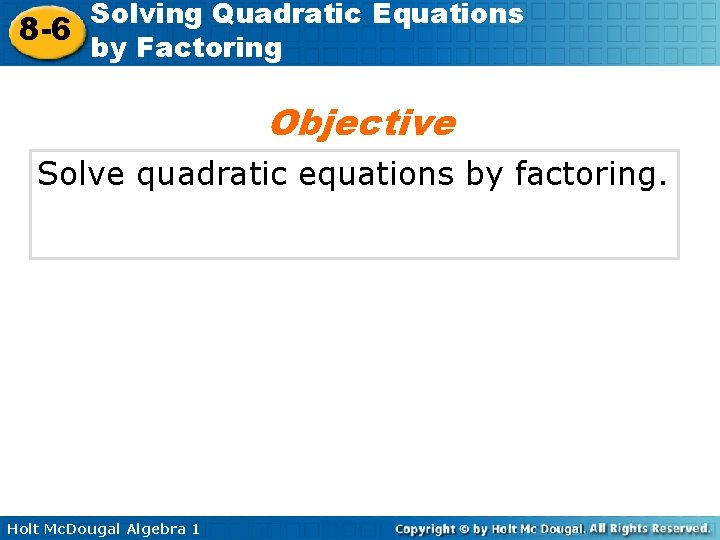Solving Quadratic Equations 8 -6 by Factoring Objective Solve quadratic equations by factoring. Holt Mc. Dougal Algebra 1Solving Quadratic Equations 8 -6 by Factoring If a quadratic equation is written in standard form, ax 2 + bx + c = 0, then to solve the equation, you may need to factor before using the Zero Product Property. Holt Mc. Dougal Algebra 1Solving Quadratic Equations 8 -6 by Factoring Example 2 A: Solving Quadratic Equations by Factoring Solve the quadratic equation by factoring. Check your answer. x 2 – 6 x + 8 = 0 (x – 4)(x – 2) = 0 Factor the trinomial. x – 4 = 0 or x – 2 = 0 Use the Zero Product Property. x = 4 or x = 2 Solve each equation. The solutions are 4 and 2. Check x 2 – 6 x + 8 = 0 (4)2 – 6(4) + 8 0 (2)2 – 6(2) + 8 0 16 – 24 + 8 0 4 – 12 + 8 0 0 0 Holt Mc. Dougal Algebra 1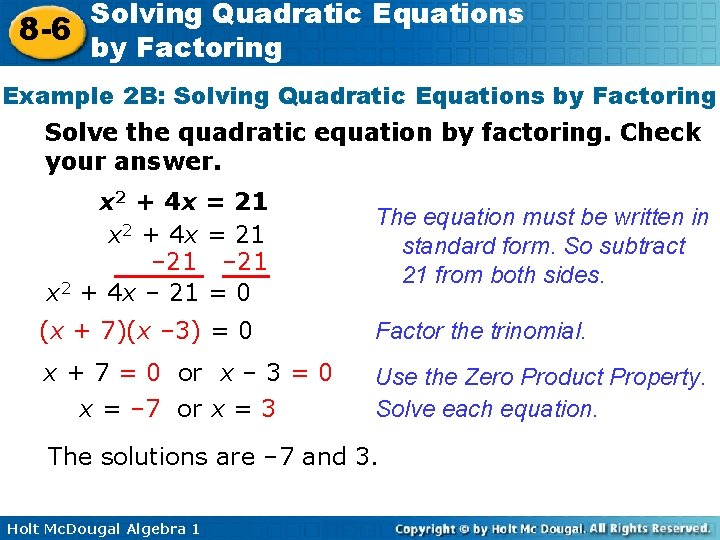Solving Quadratic Equations 8 -6 by Factoring Example 2 B: Solving Quadratic Equations by Factoring Solve the quadratic equation by factoring. Check your answer. x 2 + 4 x = 21 – 21 x 2 + 4 x – 21 = 0 The equation must be written in standard form. So subtract 21 from both sides. (x + 7)(x – 3) = 0 Factor the trinomial. x + 7 = 0 or x – 3 = 0 x = – 7 or x = 3 Use the Zero Product Property. Solve each equation. The solutions are – 7 and 3. Holt Mc. Dougal Algebra 1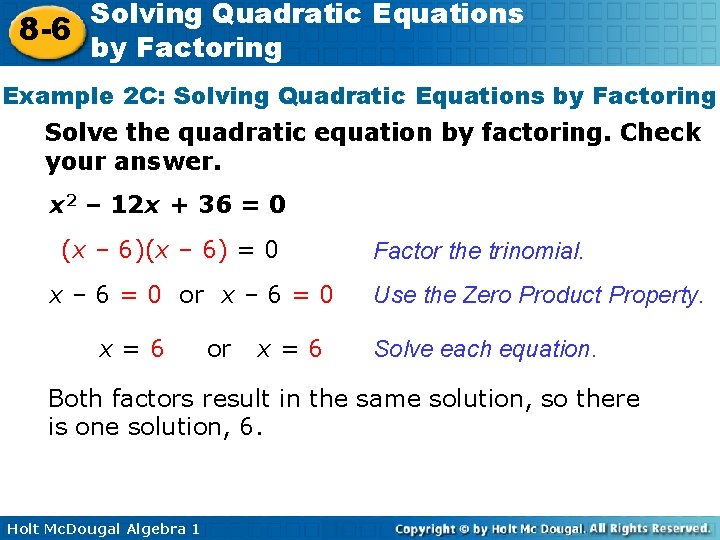Solving Quadratic Equations 8 -6 by Factoring Example 2 C: Solving Quadratic Equations by Factoring Solve the quadratic equation by factoring. Check your answer. x 2 – 12 x + 36 = 0 (x – 6) = 0 x – 6 = 0 or x – 6 = 0 x=6 or x=6 Factor the trinomial. Use the Zero Product Property. Solve each equation. Both factors result in the same solution, so there is one solution, 6. Holt Mc. Dougal Algebra 1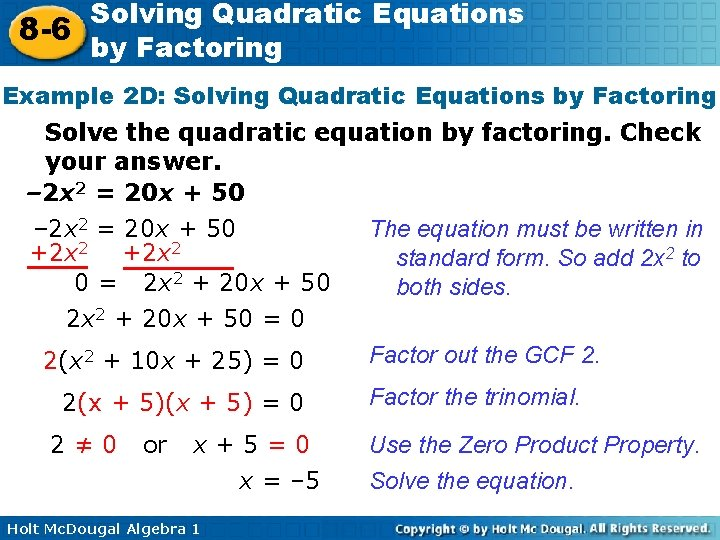Solving Quadratic Equations 8 -6 by Factoring Example 2 D: Solving Quadratic Equations by Factoring Solve the quadratic equation by factoring. Check your answer. – 2 x 2 = 20 x + 50 The equation must be written in – 2 x 2 = 20 x + 50 +2 x 2 standard form. So add 2 x 2 to 0 = 2 x 2 + 20 x + 50 both sides. 2 x 2 + 20 x + 50 = 0 Factor out the GCF 2. 2(x 2 + 10 x + 25) = 0 2(x + 5) = 0 2≠ 0 or x+5=0 x = – 5 Holt Mc. Dougal Algebra 1 Factor the trinomial. Use the Zero Product Property. Solve the equation.Solving Quadratic Equations 8 -6 by Factoring Example 2 D Continued Solve the quadratic equation by factoring. Check your answer. – 2 x 2 = 20 x + 50 Check – 2 x 2 = 20 x + 50 – 2(– 5)2 – 50 Holt Mc. Dougal Algebra 1 20(– 5) + 50 – 100 + 50 – 50 Substitute – 5 into the original equation.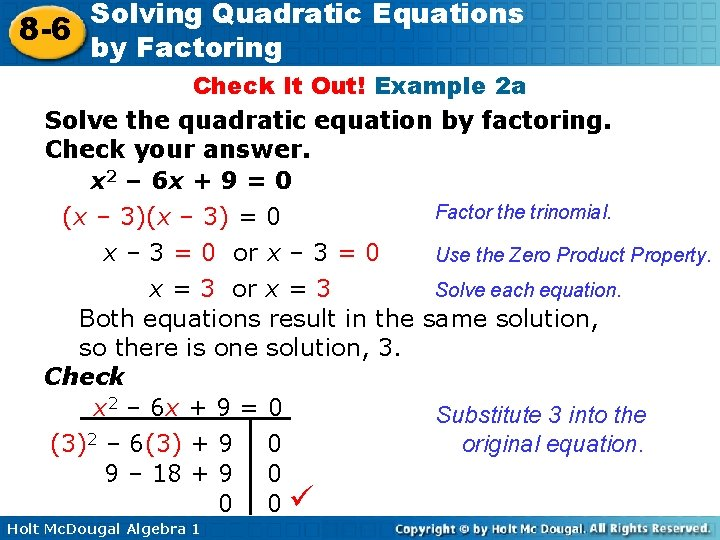Solving Quadratic Equations 8 -6 by Factoring Check It Out! Example 2 a Solve the quadratic equation by factoring. Check your answer. x 2 – 6 x + 9 = 0 Factor the trinomial. (x – 3) = 0 x – 3 = 0 or x – 3 = 0 Use the Zero Product Property. Solve each equation. x = 3 or x = 3 Both equations result in the same solution, so there is one solution, 3. Check x 2 – 6 x + 9 = 0 Substitute 3 into the original equation. (3)2 – 6(3) + 9 0 9 – 18 + 9 0 0 0 Holt Mc. Dougal Algebra 1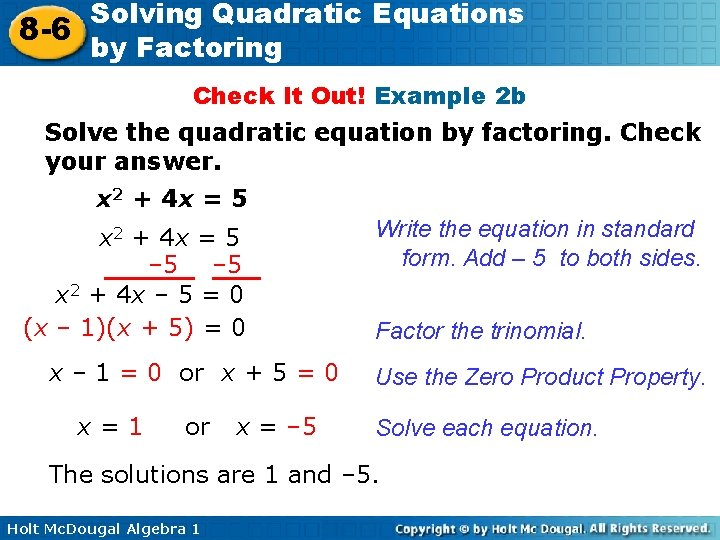Solving Quadratic Equations 8 -6 by Factoring Check It Out! Example 2 b Solve the quadratic equation by factoring. Check your answer. x 2 + 4 x = 5 – 5 x 2 + 4 x – 5 = 0 (x – 1)(x + 5) = 0 x – 1 = 0 or x + 5 = 0 x=1 or x = – 5 Write the equation in standard form. Add – 5 to both sides. Factor the trinomial. Use the Zero Product Property. Solve each equation. The solutions are 1 and – 5. Holt Mc. Dougal Algebra 1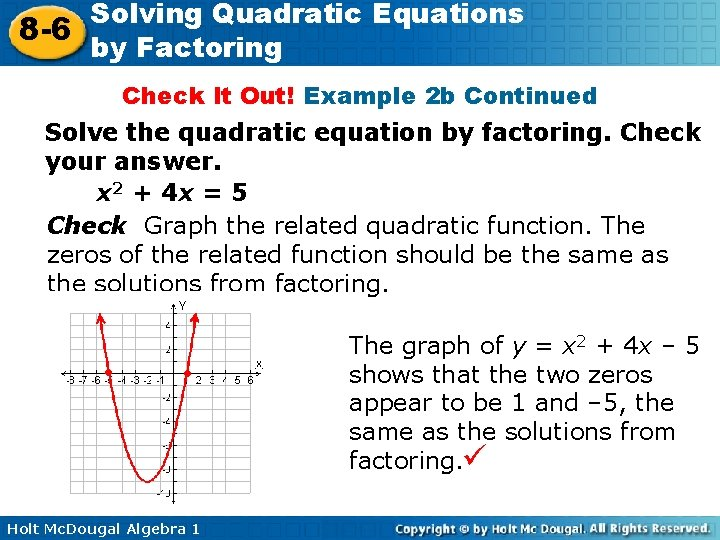Solving Quadratic Equations 8 -6 by Factoring Check It Out! Example 2 b Continued Solve the quadratic equation by factoring. Check your answer. x 2 + 4 x = 5 Check Graph the related quadratic function. The zeros of the related function should be the same as the solutions from factoring. ● ● Holt Mc. Dougal Algebra 1 The graph of y = x 2 + 4 x – 5 shows that the two zeros appear to be 1 and – 5, the same as the solutions from factoring.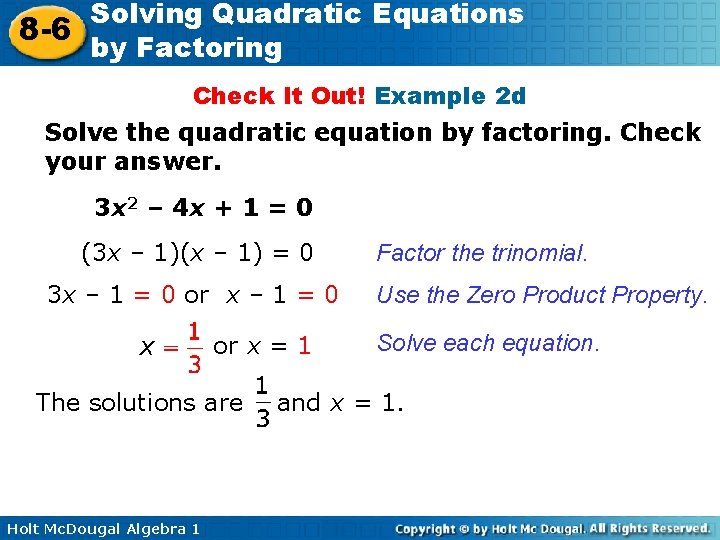Solving Quadratic Equations 8 -6 by Factoring Check It Out! Example 2 d Solve the quadratic equation by factoring. Check your answer. 3 x 2 – 4 x + 1 = 0 (3 x – 1)(x – 1) = 0 3 x – 1 = 0 or x = 1 The solutions are Holt Mc. Dougal Algebra 1 Factor the trinomial. Use the Zero Product Property. Solve each equation. and x = 1.Solving Quadratic Equations 8 -6 by Factoring Check It Out! Example 2 d Continued Solve the quadratic equation by factoring. Check your answer. 3 x 2 – 4 x + 1 = 0 Check 3 x 2 – 4 x + 1 = 0 3 – 4 +1 0 Holt Mc. Dougal Algebra 1 0 0 Check 3 x 2 – 4 x + 1 = 3(1)2 – 4(1) + 1 3– 4+1 0 0 0Solving Quadratic Equations 8 -6 by Factoring Example 3: Application The height in feet of a diver above the water can be modeled by h(t) = – 16 t 2 + 8 t + 8, where t is time in seconds after the diver jumps off a platform. Find the time it takes for the diver to reach the water. h = – 16 t 2 + 8 t + 8 0 = – 8(2 t 2 – t – 1) The diver reaches the water when h = 0. Factor out the GFC, – 8. 0 = – 8(2 t + 1)(t – 1) Factor the trinomial. 0= – 16 t 2 Holt Mc. Dougal Algebra 1 + 8 t + 8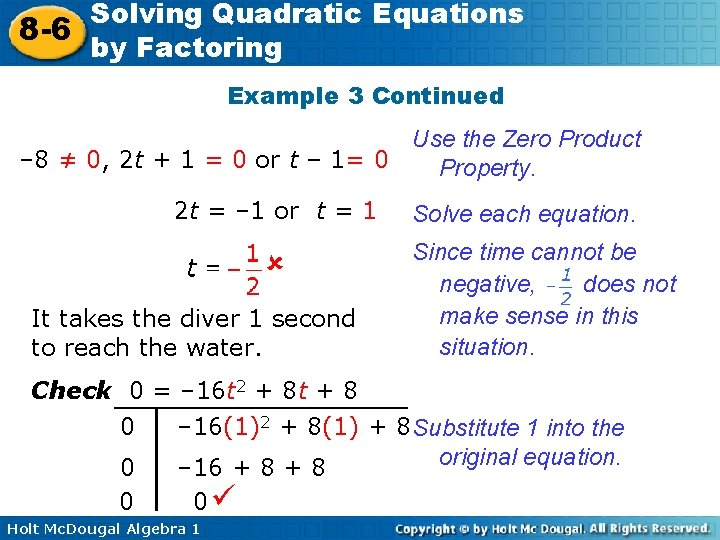Solving Quadratic Equations 8 -6 by Factoring Example 3 Continued Use the Zero Product – 8 ≠ 0, 2 t + 1 = 0 or t – 1= 0 Property. 2 t = – 1 or t = 1 It takes the diver 1 second to reach the water. Solve each equation. Since time cannot be negative, does not make sense in this situation. Check 0 = – 16 t 2 + 8 t + 8 0 – 16(1)2 + 8(1) + 8 Substitute 1 into the original equation. 0 – 16 + 8 0 0 Holt Mc. Dougal Algebra 1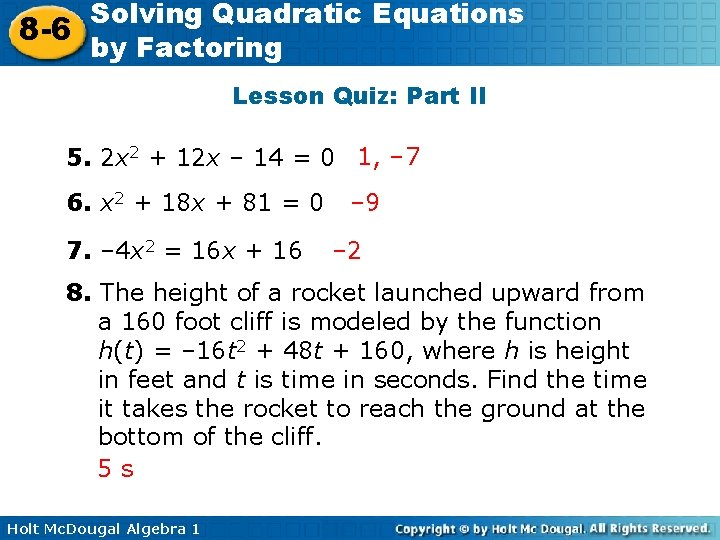Solving Quadratic Equations 8 -6 by Factoring Lesson Quiz: Part II 5. 2 x 2 + 12 x – 14 = 0 1, – 7 6. x 2 + 18 x + 81 = 0 7. – 4 x 2 = 16 x + 16 – 9 – 2 8. The height of a rocket launched upward from a 160 foot cliff is modeled by the function h(t) = – 16 t 2 + 48 t + 160, where h is height in feet and t is time in seconds. Find the time it takes the rocket to reach the ground at the bottom of the cliff. 5 s Holt Mc. Dougal Algebra 1